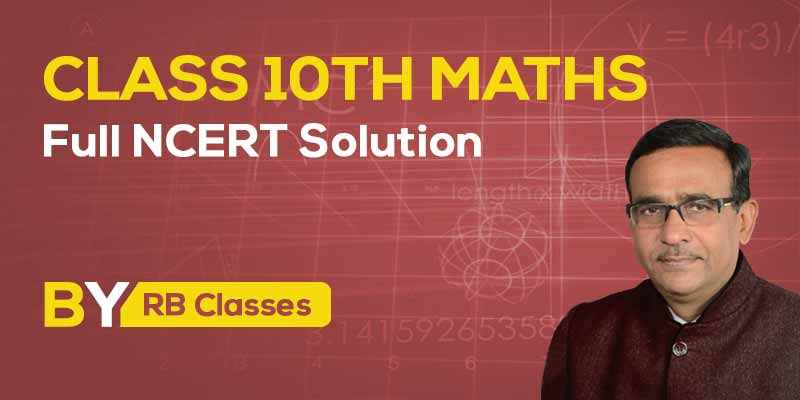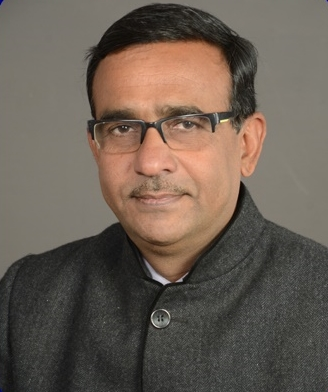Teach on Vidyakul Log in Sign up
Menu## by R B Gautam

5 (510 Users)
Class 10 , Maths
Board: CBSE & State Boards , Language: Hinglish

Mode- Online
Videos- 69
Study Notes- 34
Validity- Till 31 Mar 2020
Language- Hinglish
No. of Views- Unlimited

## WHAT WILL I LEARN?

What will Student get?

• Class 10th Maths Full NCERT Solution
• Chapter wise solution
• Important Questions.

## Curriculum

10th Maths Ch.1 Real Numbers

• Concept of Euclid’s Division Lemma and algorithm Demo Concept of Euclid’s Division Lemma and algorithm Demo
• Q1.Using Euclid’s algorithm, find the HCF of 240 and 228.
• Q3. Using Euclid’s division algorithm finds the HCF of 1656 and 4025.
• Q4.Find the HCF by Euclid’s division algorithm of the numbers 92690, 7378 and 7161. Demo Q4.Find the HCF by Euclid’s division algorithm of the numbers 92690, 7378 and 7161. Demo
• Q5.Obtain the HCF of 420 and 272 by using Euclid’s division algorithm and verify the same by using fundamental theorem of arithmetic.
• Q6.Find the HCF of 180, 252 and 324 using Euclid’s division lemma.
• Q7.Find the greatest number that will divide 445, 572 and 699 leaving remainders 4,5 and 6 respectively .
• Q8.Find HCF of 90 and 126 by Euclid’s division algorithm. Also, find their LCM and verify that LCM × HCF = Product of two numbers.
• Q9.If d is the HCF of 45 and 27, find x and y , satisfying d = 45 x + 27 y .
• Q10.If d is the HCF of 56 and 72, find x and y satisfying d = 56 x + 72 y .
• Q11.Find HCF of 81 and 237 and express it as a linear combination of 81 and 237 i.e. , HCF (81, 237) = 81x+237 y for some x and y.
• Q12.Show that one and only one out of n, n+2 or n + 4 is divisible by 3 , where n is any positive integers .
• Q13.Write whether the square of any positive integer can be of the form 3m + 2 , where m is a natural number. Justify your answer.
• Q14.Show that the square of any positive integer is of the form 4m or 4m + 1, where m is any integer.
• Q15.Use Euclid division lemma to show that the square of any positive integer cannot be of the form 5m+ 2 or 5m + 3 for integer m.
• Q16.Use Euclid’s division lemma to show that the cube of any positive integer is either of the form 9m or 9m + 1 or 9m + 8.
• Q17.Show that any positive odd integer is of the form 6q+1 or 6q+3 or 6q+5, where q is some integer.
• Q18.Prove that n2 – n is divisible by 2 for every positive integer n.
• Q19.For any positive integer n, prove that n3 – n is divisible by 6.
• Q20. In a school, the duration of a period in junior sections is 40 minutes and senior section is 1 hour. If the first bell for each section rings at 9: 00 a. m, when will the two bells ring together again?
• Q21.Two tankers contain 620 liters and 840 liters of diesel respectively. Find the maximum capacity of a container which can measure the diesel of both the tankers in exact number of times.
• Q22.Concept of Fundamental Theorem of Arithmetic.
• Q23.Find the missing number in the following factorization.
• Q24.Complete the following factor tree and find the composite number x and y.
• Q25.Find the smallest natural number by which 1200 should be multiplied so that the square root of the product is a rational number.
• Q26.Check whether (i) (15 )n (ii) (4 )n (iii) ( 30 )n can end with the digit zero.
• Q27.Explain whether 3 × 12 × 101+4 is a prime number or a composite number.
• Q28.Write the HCF and LCM of the smallest odd composite number and the smallest odd prime number. If an odd number p divides q 2, then will it divide q3, also? Explain.
• Q29.If p is a prime number then prove that √p is irrational.
• Q30.Prove that √(P )+ √q is irrational, where p and q are primes.
• Q31.Prove that √5 is an irrational number and hence show that 2- √5 is also an irrational number.
• Q32.Is (π- 22/7) a rational number, an irrational number or zero?
• Q33.Can two numbers have 15 as their HCF and 175 as their LCM? Give reasons.
• Q34.The LCM of two numbers is 14 times their HCF . The sum of LCM and HCF is 600. If one number is 280, then find the other number .

10th Maths Ch.2 Polynomials

• 1. NCERT EXEMPLAR Exercise 2.1 Q1
• 2. NCERT EXEMPLAR Exercise 2.1 Q2
• 3. NCERT EXEMPLAR Exercise 2.1 Q3
• 4. NCERT EXEMPLAR Exercise 2.1 Q4
• 5. NCERT EXEMPLAR Exercise 2.1 Q5
• 6. NCERT EXEMPLAR Exercise 2.1 Q6
• 7. NCERT EXEMPLAR Exercise 2.1 Q7
• 8. NCERT EXEMPLAR Exercise 2.1 Q8
• 9. NCERT EXEMPLAR Exercise 2.1 Exam 1
• 10. NCERT EXEMPLAR Exercise 2.1 Exam 2
• 11. Important Questions, for CBSE Board Exam
• 12. Important Questions for CBSE Board Exam Q9
• 13. Find Polynomial whose zeros are given CBSE 2019 Q2
• 14. Important questions of Maths for class 10
• 15. Class 10 Maths Extra Questions
• 16. Important Questions for board exam 2019

10th Maths Ch.3- Linear Equations in Two Variables

• 1. Q1 Solve the pair of linear equations by graphically
• 2. Q2 Distance, Speed & Time Word Problem
• 3. Q3 Find values of a and b if equations has infinitely many solution
• 4. Q4 Find the speed of the boat in still water and the speed of the stream
• 5. Q5 Class 10 maths linear equations
• 6. Q6 Linear equations in two variables word problems
• 7. Q7 Elimination method for solving system of equations
• 8. Pair of Linear Equations Important Questions Q1
• 9. Pair of Linear Equations(Substitution Method ) Important Questions NCERT Q2
• 10. Word problems on linear equations in two variables Important Question Q3
• 11. Linear Equations in two variables Important questions NCERT Q4
• 12. Solution of linear Equations by Cross Multiplication Method Q5
• 13. Linear Equations in two variable NCERT Q6
• 14. Find the value of a and b if Equations has infinite many solution Q7
• 15. Word Problems in Linear Equation IMP Question NCERT Q8
• 16. Linear Equations In Two Variables
• 17. Linear Equations In Two Variables ,for what value of k do the equations
• 18. Linear Equations In Two Variables, solve the system of equations graphically
• 19. Linear Equations In Two Variables , linear equations by the elimination method.

10th Maths Ch.4 Quadratic Equations

10th Maths Ch.5 Arithmetic Progressions

10th Maths Ch.6 Triangles

10th Maths Ch.7 Coordinate Geometry

10th Maths Ch.8 Introduction to Trigonometry

10th Maths Ch.9 Some Applications of Trigonometry

10th Maths Ch.10 Circles

10th Maths Ch.11 Constructions

10th Maths Ch.12 Areas Related to Circles

10th Maths Ch.13 Surface Areas and Volume

10th Maths Ch.14 Statistics

10th Maths Ch.15 Probability

## About R B Gautam### R B Gautam

Mathematics | Class 9th to 12th | 25+ Years Experience | R B Gautam Sir is a well-known for his expertise in Math’s. He is serving the society by providing students with quality content to achieve 100 out of 100 in their math’s exams. R B Gautam Sir helps students by solving and explaining major problems faced by students in their exams.

## 3999 2499

Includes 18 % GST

Mode- Online

Videos- 69

Study Notes- 34

Validity- Till 31 Mar 2020

Language- Hinglish

No. of Views- Unlimited